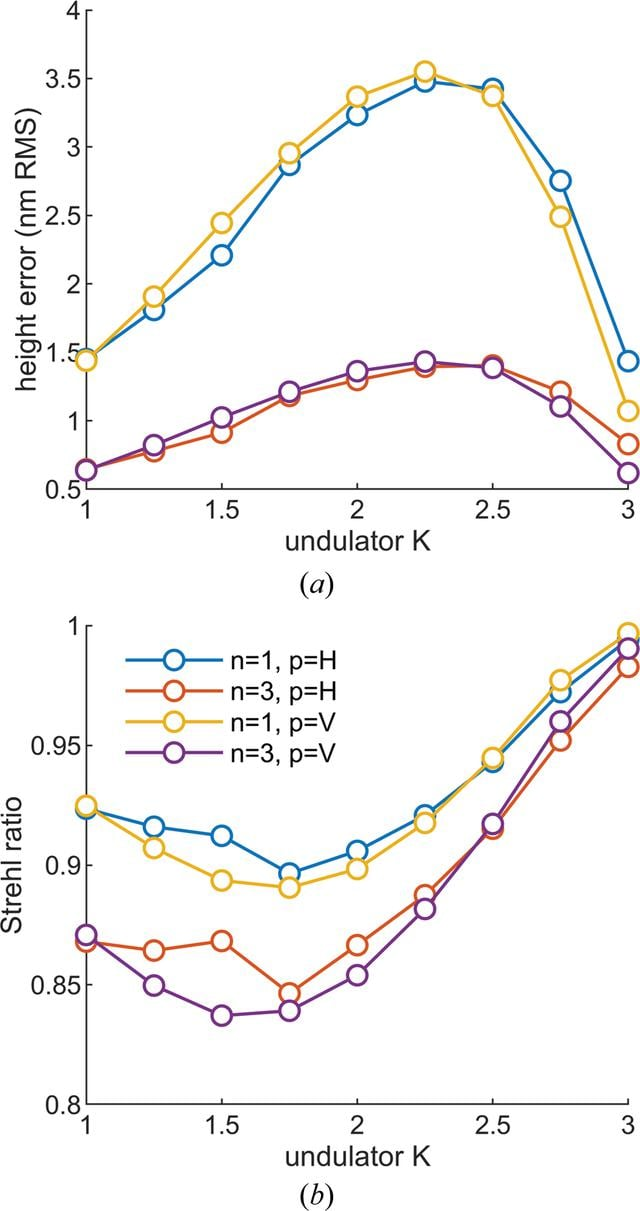disable zoom     view article Figure 10 (a) The height error and (b) the Strehl ratio as a function of undulator deflection parameter K for the first (n = 1) and third (n = 3) harmonics and the linear horizontal (p = H) and vertical (p = V) polarization modes. Note that while the distortion of the mirror depends only on the undulator K and the polarization mode, the r.m.s. window is 6σ of the spatial distribution of the photons of interest, and therefore depends on the harmonic number. The dip in the height error and the corresponding rise in the Strehl ratio at K = 1.5 for linear horizontal polarization is due to the nonlinearity of the material properties of silicon combined with the heat-load distribution.JOURNAL OFSYNCHROTRONRADIATION
ISSN: 1600-5775
Volume 27| Part 5| September 2020| Pages 1131-1140